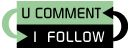## Friday, March 26, 2010

### Solving mathematical puzzles with brute-force and Perl

After talking a lot about optimizations and selecting the right algorithm, here is a little brute-force code. This particular one gives the answer to the following puzzle from Richard Wiseman's Blog (one well worth following BTW):

Can you make the number 24 with the number 5, 5, 5, and 1 (again, you cannot join the numbers together, have to use each number once and only once, and are only allowed to add, subtract, multiply or divide them)?

And here is my brute-force solution:

``````
permute(0, [5, 5, 5, 1], []);

sub permute {
my (\$partial, \$numbers, \$solution) = @_;

if (0 == scalar(@\$numbers)) {
print @\$solution, "\n" if (24 == \$partial);
}
else {
for my \$num (@\$numbers) {
my \$mynums = [];
my \$skipped = 0;
for my \$mynum (@\$numbers) {
if (\$num == \$mynum && !\$skipped) {
\$skipped = 1;
}
else {
push @\$mynums, \$mynum;
}
}

for my \$op (qw(- + * /)) {
my \$mypart = eval "\$partial \$op \$num";
my \$mysol  = [@\$solution, \$op, \$num];
permute(\$mypart, \$mynums, \$mysol);
}
}
}
}
``````

The output is not very elegant and contains a decent amount of garbage (because it considers that we have a hidden zero at the start – ie. 0*5...) and also a lot of repetition (because it doesn’t take into account that 5 5 5 1 is the same as 5 5 5 1 with the first two numbers interchanged), but in the end it gives the correct answer:

``````
... fake answers because it starts with 0 ...
*5+5*5-1
*5+5*5-1
/5+5*5-1
/5+5*5-1
*5+5*5-1
*5+5*5-1
/5+5*5-1
/5+5*5-1
*5+5*5-1
*5+5*5-1
/5+5*5-1
/5+5*5-1
... duplicate answers because of the order ...
-1/5+5*5
-1/5+5*5
-1/5+5*5
-1/5+5*5
-1/5+5*5
-1/5+5*5
``````

Also the correct interpretation of the output is to consider that each operation has a pair of parentheses around them and not reading it according to the usual mathematical rules. Having this in mind the solution becomes:

((-1/5)+5)*5 = 4.8 * 5 = 24

Brute-force FTW :-)